# LombScargle.jl¶

## Introduction¶

LombScargle.jl is a Julia package to estimate the frequency spectrum of a periodic signal with the Lomb–Scargle periodogram.

Another Julia package that provides tools to perform spectral analysis of signals is DSP.jl, but its methods require that the signal has been sampled at equally spaced times. Instead, the Lomb–Scargle periodogram enables you to analyze unevenly sampled data as well, which is a fairly common case in astronomy.

The algorithm used in this package are reported in the following papers:

 [TOW10] Townsend, R. H. D. 2010, ApJS, 191, 247 (URL: http://dx.doi.org/10.1088/0067-0049/191/2/247, Bibcode: http://adsabs.harvard.edu/abs/2010ApJS..191..247T)
 [ZK09] (1, 2, 3, 4, 5) Zechmeister, M., Kürster, M. 2009, A&A, 496, 577 (URL: http://dx.doi.org/10.1051/0004-6361:200811296, Bibcode: http://adsabs.harvard.edu/abs/2009A%26A...496..577Z)

Othe relevant papers are:

 [CMB99] (1, 2, 3) Cumming, A., Marcy, G. W., & Butler, R. P. 1999, ApJ, 526, 890 (URL: http://dx.doi.org/10.1086/308020, Bibcode: http://adsabs.harvard.edu/abs/1999ApJ...526..890C)
 [CUM04] (1, 2, 3) Cumming, A. 2004, MNRAS, 354, 1165 (URL: http://dx.doi.org/10.1111/j.1365-2966.2004.08275.x, Bibcode: http://adsabs.harvard.edu/abs/2004MNRAS.354.1165C)
 [HB86] Horne, J. H., & Baliunas, S. L. 1986, ApJ, 302, 757 (URL: http://dx.doi.org/10.1086/164037, Bibcode: http://adsabs.harvard.edu/abs/1986ApJ...302..757H)
 [LOM76] Lomb, N. R. 1976, Ap&SS, 39, 447 (URL: http://dx.doi.org/10.1007/BF00648343, Bibcode: http://adsabs.harvard.edu/abs/1976Ap%26SS..39..447L)
 [SCA82] Scargle, J. D. 1982, ApJ, 263, 835 (URL: http://dx.doi.org/10.1086/160554, Bibcode: http://adsabs.harvard.edu/abs/1982ApJ...263..835S)
 [SS10] Sturrock, P. A., & Scargle, J. D. 2010, ApJ, 718, 527 (URL: http://dx.doi.org/10.1088/0004-637X/718/1/527, Bibcode: http://adsabs.harvard.edu/abs/2010ApJ...718..527S)

## Installation¶

LombScargle.jl is available for Julia 0.4 and later versions, and can be installed with Julia built-in package manager. In a Julia session run the command

julia> Pkg.add("LombScargle")


You may need to update your package list with Pkg.update() in order to get the latest version of LombScargle.jl.

## Usage¶

After installing the package, you can start using it with

using LombScargle


The module defines a new LombScargle.Periodogram data type, which, however, is not exported because you will most probably not need to directly manipulate LombScargle.Periodogram objects. This data type holds both the frequency and the power vectors of the periodogram.

The main function provided by the package is lombscargle:

lombscargle(times::AbstractVector{Real}, signal::AbstractVector{Real})

which returns a LombScargle.Periodogram. The mandatory arguments are:

• times: the vector of observation times
• signal: the vector of observations associated with times

All these vectors must have the same length. The complete syntax of lombscargle is the following:

lombscargle(times::AbstractVector{Real}, signal::AbstractVector{Real},
errors::AbstractVector{Real}=ones(signal);
normalization::AbstractString="standard",
noise_level::Real=1.0,
center_data::Bool=true, fit_mean::Bool=true,
samples_per_peak::Integer=5,
nyquist_factor::Integer=5,
minimum_frequency::Real=NaN,
maximum_frequency::Real=NaN,
frequencies::AbstractVector{Real}=
autofrequency(times,
samples_per_peak=samples_per_peak,
nyquist_factor=nyquist_factor,
minimum_frequency=minimum_frequency,
maximum_frequency=maximum_frequency))


In addition to the above mentioned mandatory argument, there is an optional argument:

• errors: the uncertainties associated to each signal point

Also errors must have the same length as times and signal.

Optional keyword arguments are:

• normalization: how to normalize the periodogram. Valid choices are: "standard", "model", "log", "psd", "Scargle", "HorneBaliunas", "Cumming". See Normalization section for details
• noise_level: the noise level used to normalize the periodogram when normalization is set to "Scargle"
• fit_mean: if true, fit for the mean of the signal using the Generalised Lomb–Scargle algorithm (see [ZK09]). If this is false, the original algorithm by Lomb and Scargle will be employed (see [TOW10]), which does not take into account a non-null mean and uncertainties for observations
• center_data: if true, subtract the mean of signal from signal itself before performing the periodogram. This is especially important if fit_mean is false
• frequencies: the frequecy grid (not angular frequencies) at which the periodogram will be computed, as a vector. If not provided, it is automatically determined with LombScargle.autofrequency function, which see. See below for other available keywords that can be used to adjust the frequency grid without directly setting frequencies

In addition, you can use all optional keyword arguments of LombScargle.autofrequency function in order to tune the frequencies vector without calling the function:

• samples_per_peak: the approximate number of desired samples across the typical peak
• nyquist_factor: the multiple of the average Nyquist frequency used to choose the maximum frequency if maximum_frequency is not provided
• minimum_frequency: if specified, then use this minimum frequency rather than one chosen based on the size of the baseline
• maximum_frequency: if specified, then use this maximum frequency rather than one chosen based on the average Nyquist frequency

The frequency grid is determined by following prescriptions given at https://jakevdp.github.io/blog/2015/06/13/lomb-scargle-in-python/ and uses the same keywords names adopted in Astropy.

If the signal has uncertainties, the signal vector can also be a vector of Measurement objects (from Measurements.jl package), in which case you don’t need to pass a separate errors vector for the uncertainties of the signal. You can create arrays of Measurement objects with the measurement function, see Measurements.jl manual at http://measurementsjl.readthedocs.io/ for more details.

### Normalization¶

By default, the periodogram $$p(f)$$ is normalized so that it has values in the range $$0 \leq p(f) \leq 1$$, with $$p = 0$$ indicating no improvement of the fit and $$p = 1$$ a “perfect” fit (100% reduction of $$\chi^2$$ or $$\chi^2 = 0$$). This is the normalization suggested by [LOM76] and [ZK09], and corresponds to the "standard" normalization in lombscargle() function. [ZK09] wrote the formula for the power of the periodogram at frequency $$f$$ as

$$p(f) = \frac{1}{YY}\left[\frac{YC^2_{\tau}}{CC_{\tau}} + \frac{YS^2_{\tau}}{SS_{\tau}}\right]$$

See the paper for details. The other normalizations for periodograms $$P(f)$$ are calculated from this one. In what follows, $$N$$ is the number of observations.

• "model": $$P(f) = \frac{p(f)}{1 - p(f)}$$
• "log": $$P(f) = -\log(1 - p(f))$$
• "psd": $$P(f) = \frac{1}{2}\left[\frac{YC^2_{\tau}}{CC_{\tau}} + \frac{YS^2_{\tau}}{SS_{\tau}}\right] = p(f) \frac{YY}{2}$$
• "Scargle": $$P(f) = \frac{p(f)}{\text{noise level}}$$ This normalization can be used when you know the noise level (expected from the a priori known noise variance or population variance), but this isn’t usually the case. See [SCA82]
• "HorneBaliunas": $$P(f) = \frac{N - 1}{2} p(f)$$ This is like the "Scargle" normalization, where the noise has been estimated for Gaussian noise to be $$(N - 1)/2$$. See [HB86]
• If the data contains a signal or if errors are under- or overestimated or if intrinsic variability is present, then $$(N-1)/2$$ may not be a good uncorrelated estimator for the noise level. [CMB99] suggested to estimate the noise level a posteriori with the residuals of the best fit and normalised the periodogram as: $$P(f) = \frac{N - 3}{2} \frac{p(f)}{1 - p(f_{\text{best}})}$$ This is the "Cumming" normalization option

### Access Frequency Grid and Power Spectrum of the Periodogram¶

power(p::Periodogram)
freq(p::Periodogram)
freqpower(p::Periodogram)

lombscargle() function return a LombScargle.Periodogram object, but you most probably want to use the frequency grid and the power spectrum. You can access these vectors with freq and power functions, just like in DSP.jl package. If you want to get the 2-tuple (freq(p), power(p)) use the freqpower function.

### Find Highest Power and Associated Frequencies¶

findmaxpower(p::Periodogram)
findmaxfreq(p::Periodogram, threshold::Real=findmaxpower(p))

Once you compute the periodogram, you usually want to know which are the frequencies with highest power. To do this, you can use the findmaxfreq. It returns the vector of frequencies with the highest power in the periodogram p. If a second argument threshold is provided, return the frequencies with power larger than or equal to threshold. The value of the highest power of a periodogram can be calculated with the findmaxpower function.

### False-Alarm Probability¶

prob(P::Periodogram, p_0::Real)
probinv(P::Periodogram, prob::Real)
fap(P::Periodogram, p_0::Real)
fapinv(P::Periodogram, fap::Real)

Noise in the data produce fluctuations in the periodogram that will present several local peaks, but not all of them related to real periodicities. The significance of the peaks can be tested by calculating the probability that its power can arise purely from noise. The higher the value of the power, the lower will be this probability.

Note

[CMB99] showed that the different normalizations result in different probability functions. LombScargle.jl can calculate the probability (and the false-alarm probability) only for the normalizations reported by [ZK09], that are "standard", "Scargle", "HorneBaliunas", and "Cumming".

The probability $$\text{Prob}(p > p_{0})$$ that the periodogram power $$p$$ can exceed the value $$p_{0}$$ can be calculated with the prob function, whose first argument is the periodogram and the second one is the $$p_{0}$$ value. The function probinv is its inverse: it takes the probability as second argument and returns the corresponding $$p_{0}$$ value.

Here are the probability functions for each normalization supported by LombScargle.jl:

• "standard" ($$p \in [0, 1]$$): $$\text{Prob}(p > p_{0}) = (1 - p_{0})^{(N - 3)/2}$$
• "Scargle" ($$p \in [0, \infty)$$): $$\text{Prob}(p > p_{0}) = \exp(-p_{0})$$
• "HorneBaliunas" ($$p \in [0, (N - 1)/2]$$): $$\text{Prob}(p > p_{0}) = \left(1 - \frac{2p_{0}}{N - 1}\right)^{(N - 3)/2}$$
• "Cumming" ($$p \in [0, \infty)$$): $$\text{Prob}(p > p_{0}) = \left(1 + \frac{2p_{0}}{N - 3}\right)^{-(N - 3)/2}$$

As explained by [SS10], «the term “false-alarm probability” denotes the probability that at least one out of $$M$$ independent power values in a prescribed search band of a power spectrum computed from a white-noise time series is expected to be as large as or larger than a given value». LombScargle.jl provides the fap function to calculate the false-alarm probability (FAP) of a given power in a periodogram. Its first argument is the periodogram, the second one is the value $$p_{0}$$ of the power of which you want to calculate the FAP. The function fap uses the formula

$$\text{FAP} = 1 - (1 - \text{Prob}(p > p_{0}))^M$$

where $$M$$ is the number of independent frequencies estimated with $$M = T \cdot \Delta f$$, being $$T$$ the duration of the observations and $$\Delta f$$ the width of the frequency range in which the periodogram has been calculated (see [CUM04]). The function fapinv is the inverse of fap: it takes as second argument the value of the FAP and returns the corresponding value $$p_{0}$$ of the power.

The detection threshold $$p_{0}$$ is the periodogram power corresponding to some (small) value of $$\text{FAP}$$, i.e. the value of $$p$$ exceeded due to noise alone in only a small fraction $$\text{FAP}$$ of trials. An observed power larger than $$p_{0}$$ indicates that a signal is likely present (see [CUM04]).

Caution

Some authors stressed that this method to calculate the false-alarm probability is not completely reliable. A different approach to calculate the false-alarm probability is to perform Monte Carlo or bootstrap simulations in order to determine how often a certain power level $$p_{0}$$ is exceeded just by chance (see [CMB99], [CUM04], and [ZK09]).

## Examples¶

Here is an example of a noisy periodic signal ($$\sin(\pi t) + 1.5\cos(2\pi t)$$) sampled at unevenly spaced times.

using LombScargle
ntimes = 1001
# Observation times
t = linspace(0.01, 10pi, ntimes)
# Randomize times
t += step(t)*rand(ntimes)
# The signal
s = sinpi(t) + 1.5cospi(2t) + rand(ntimes)
pgram = lombscargle(t, s)


You can plot the result, for example with PyPlot package. Use freqpower function to get the frequency grid and the power of the periodogram as a 2-tuple.

using PyPlot
plot(freqpower(pgram)...)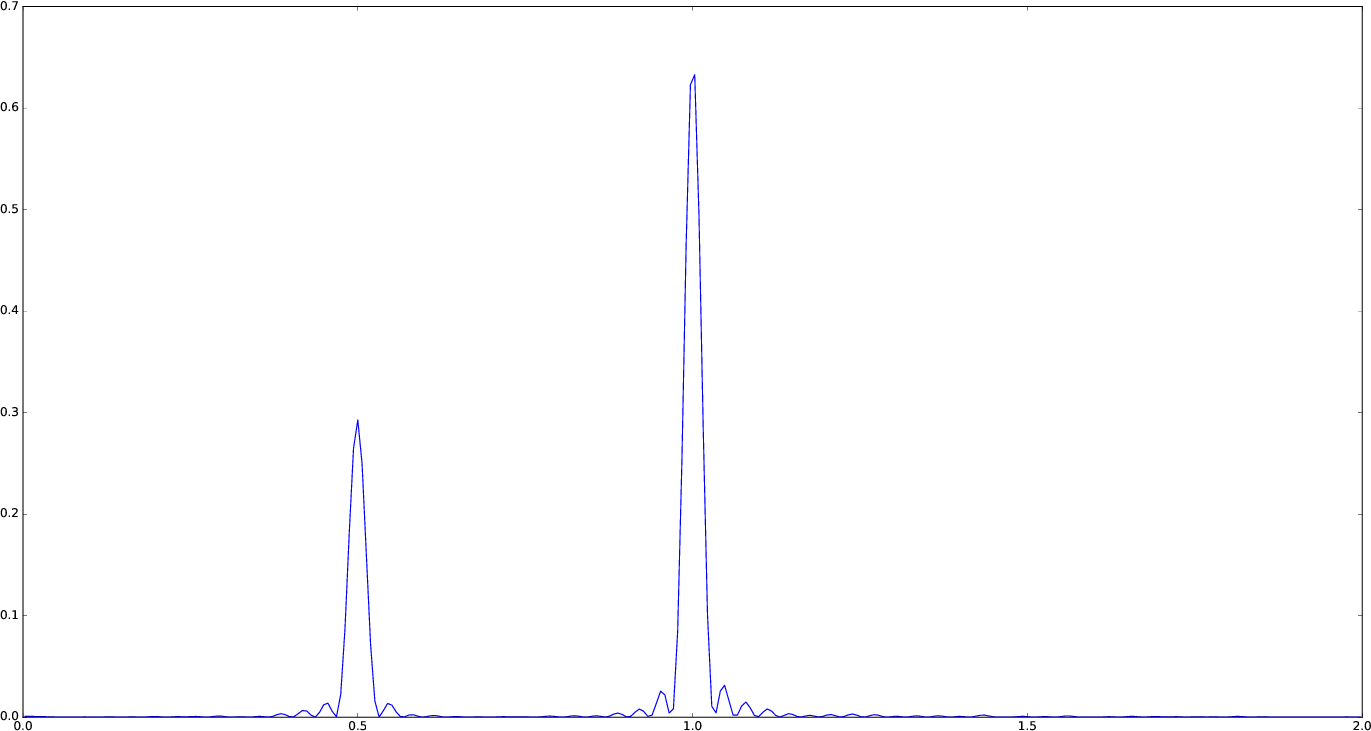Caution

If you use original Lomb–Scargle algorithm (fit_mean=false keyword to lombscargle() function) without centering the data (center_data=false) you can get inaccurate results. For example, spurious peaks at low frequencies can appear and the real peaks lose power:

plot(freqpower(lombscargle(t, s, fit_mean=false, center_data=false))...)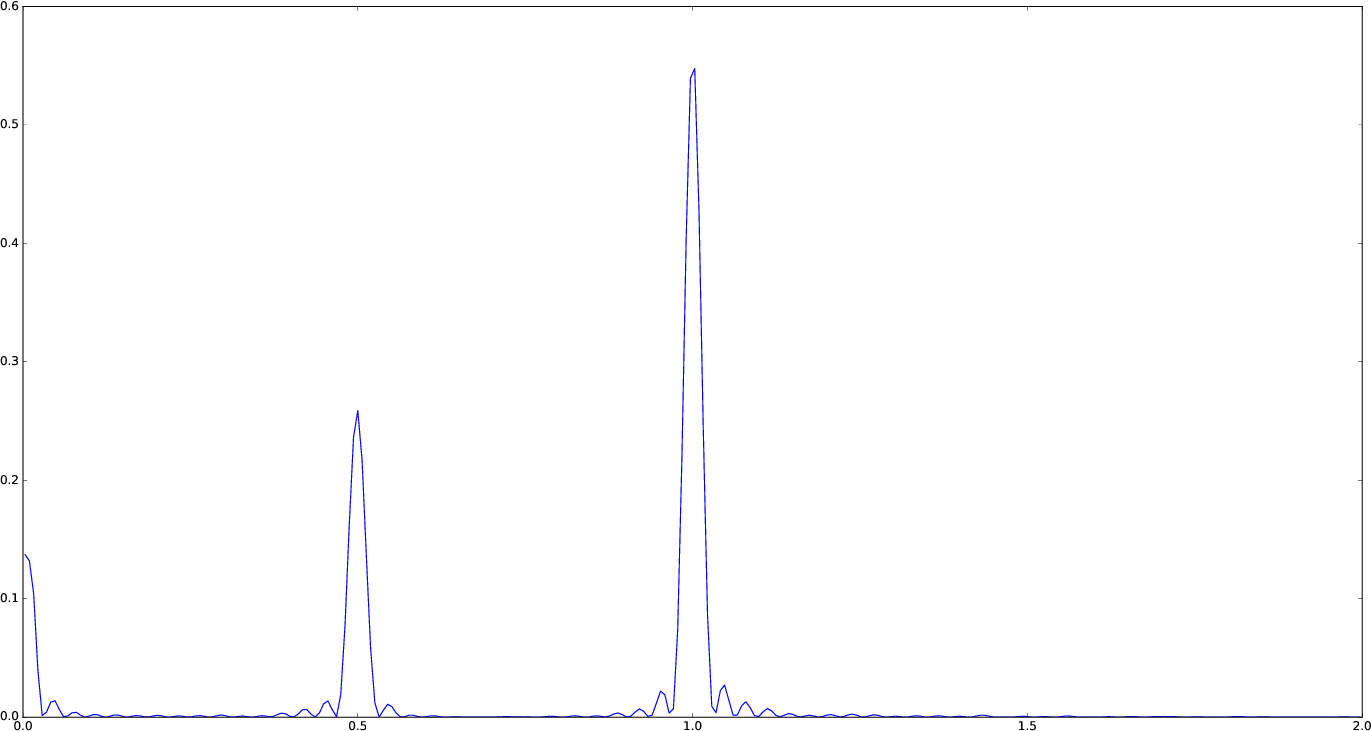Tip

You can tune the frequency grid with appropriate keywords to lombscargle() function. For example, in order to increase the sampling increase samples_per_peak, and set maximum_frequency to lower values in order to narrow the frequency range:

plot(freqpower(lombscargle(t, s, samples_per_peak=20, maximum_frequency=1.5))...)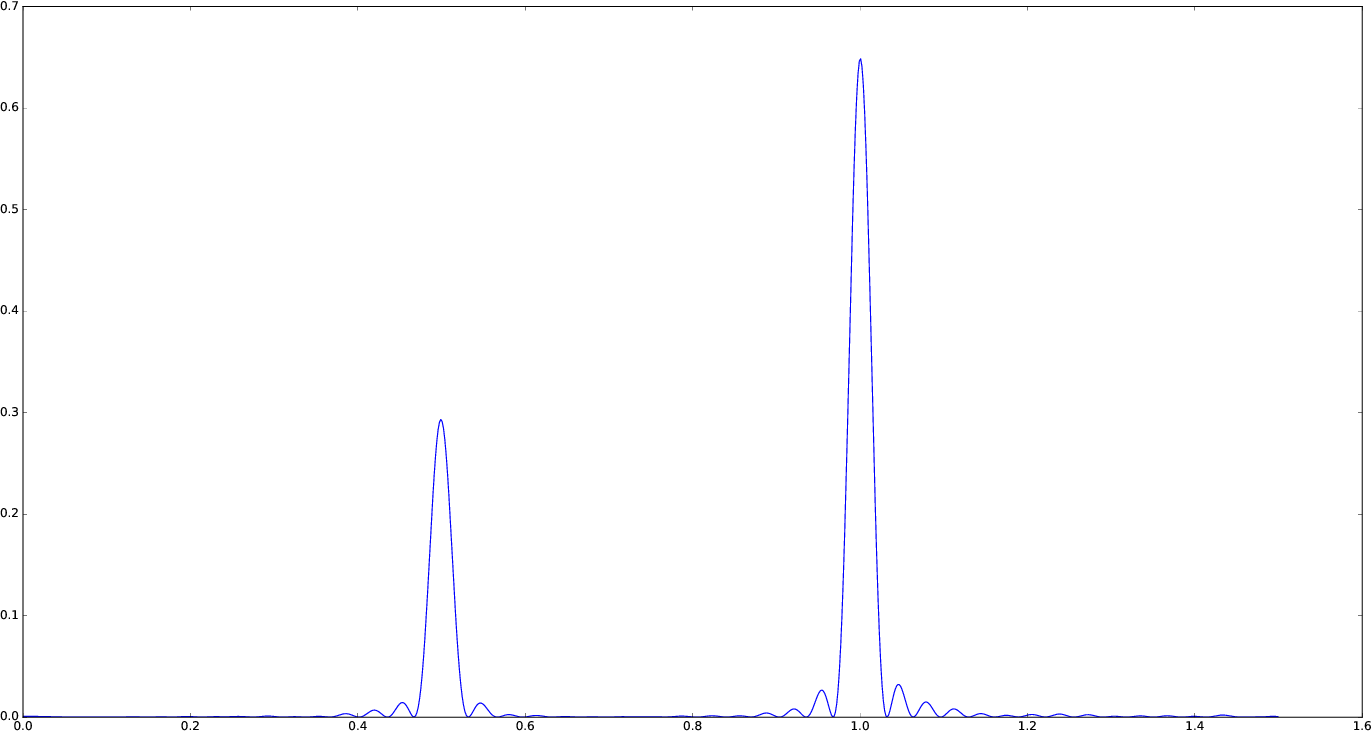If you simply want to use your own frequency grid, directly set the frequencies keyword:

plot(freqpower(lombscargle(t, s, frequencies=0.001:1e-3:1.5))...)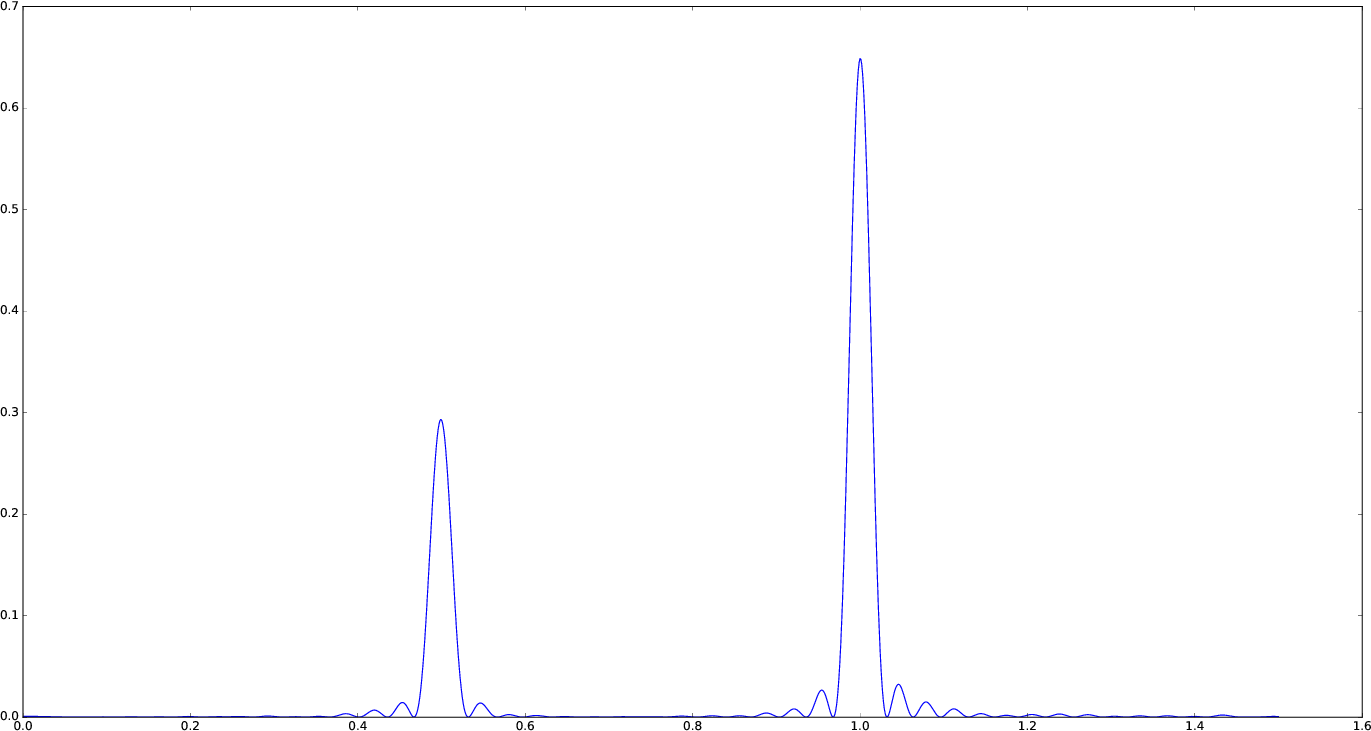### Signal with Uncertainties¶

The generalised Lomb–Scargle periodogram (used when the fit_mean optional keyword is true) is able to handle a signal with uncertainties, and they will be used as weights in the algorithm. The uncertainties can be passed either as the third optional argument errors to lombscargle() or by providing this function with a signal vector of type Measurement (from Measurements.jl package).

using Measurements, PyPlot
ntimes = 1001
t = linspace(0.01, 10pi, ntimes)
s = sinpi(2t)
errors = rand(0.1:1e-3:4.0, ntimes)
plot(freqpower(lombscargle(t, s, errors, maximum_frequency=1.5))...)
plot(freqpower(lombscargle(t, measurement(s, errors), maximum_frequency=1.5))...)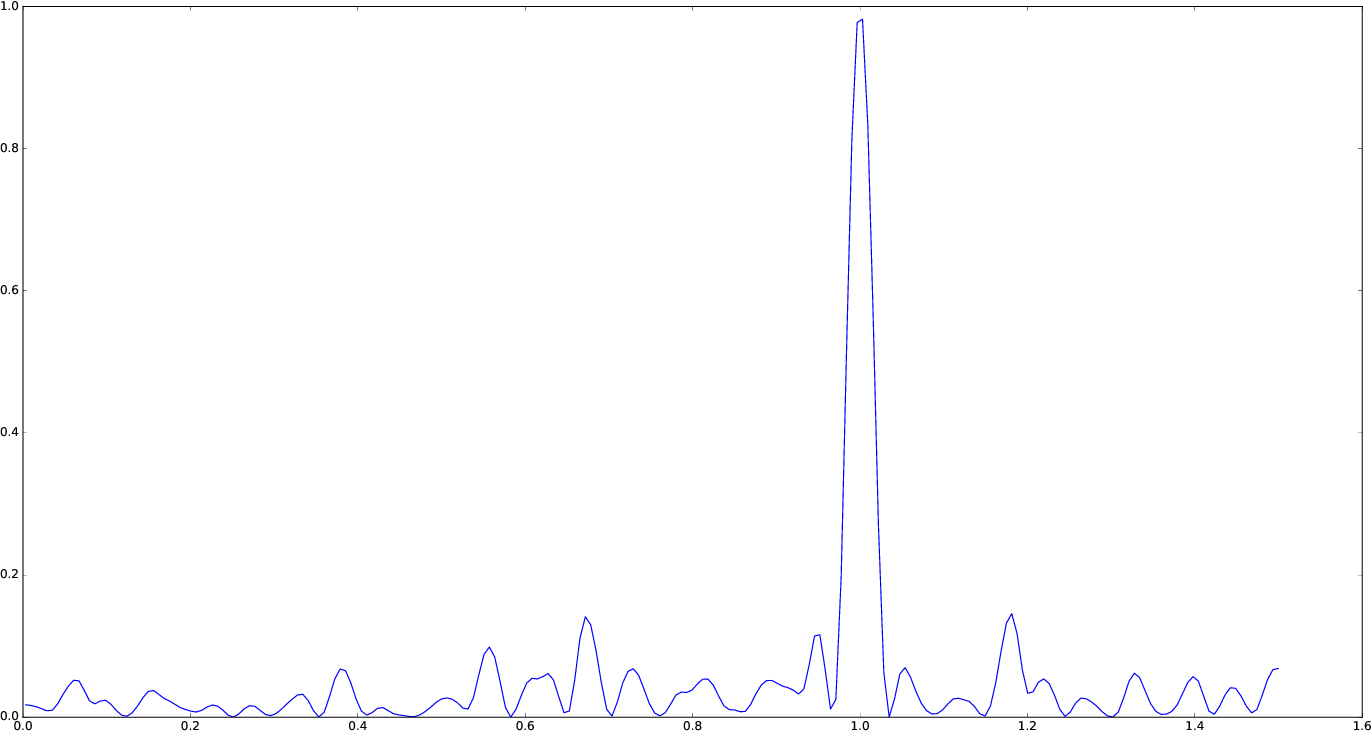### findmaxfreq and findmaxpower Functions¶

findmaxfreq function tells you the frequencies with the highest power in the periodogram (and you can get the period by taking its inverse):

t = linspace(0, 10, 1001)
s = sinpi(2t)
p = lombscargle(t, s)
1.0./findmaxfreq(p) # Period with highest power
# => 1-element Array{Float64,1}:
#     0.00502487


This peak is at high frequency, very far from the expected value of the period of 1. In order to find the real peak, you can either narrow the frequency range in order to exclude higher armonics, or pass the threshold argument to findmaxfreq. You can use findmaxpower to discover the highest power in the periodogram:

findmaxpower(p)
# => 0.9712085205753647
1.0./findmaxfreq(p, 0.97)
# => 5-element Array{Float64,1}:
#     1.0101
#     0.0101
#     0.00990197
#     0.00502487
#     0.00497537


The first peak is the real one, the other double peaks appear at higher armonics.

Tip

Usually plotting the periodogram can give you a clue of what’s going on.

## Development¶

The package is developed at https://github.com/giordano/LombScargle.jl. There you can submit bug reports, make suggestions, and propose pull requests.

### History¶

The ChangeLog of the package is available in NEWS.md file in top directory.

The LombScargle.jl package is licensed under the MIT “Expat” License. The original author is Mosè Giordano.